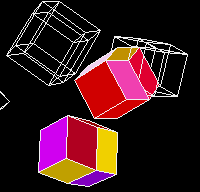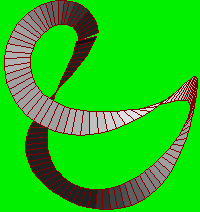#Rudy Rucker's Ten Mathematics FavoritesReprinted from The SF Book of Lists by Maxim Jakubowski and Malcolm Edwards (Berkley, 1983)

1. Archytas' Duplication of the Cube
The Greeks were interested in how one might go about constructing a line segment of length equal to the cube root of two. This is impossible with ruler and compass alone, but Archytas found a way of doing it by looking at the common intersection of cone, cylinder and torus.

2. Hinton's Tesseract
Charles H.Hinton coined the word "tesseract", for a four-dimensional hypercube. By playing with a set of 81 small colored cubes he learned how to visualize it. He also invented a gun for shooting baseballs.

3. The Klein Bottle
This was the idea of Felix Klein, a 19th-century German mathemetician. Many people have heard of it, but few really understand it. In a nutshell, the Klein bottle is to four-dimensional space as the Möbius strip is to three-dimensional space.

4. Poincaré's Model of the Hyperbolic Plane
In order to prove the consistency of non-Euclidean geometry, Poincaré constructed this mathematical model. Here we have an infinite space squeezed inside the bounds of a circle. Someone who starts out from the center of the circle shrinks in such a way that he can never reach the circumference.

5. The Grandi Series
This is the infinite series 1 - 1 + 1 - 1 + . . . Viewed in one way, the Grandi series adds up to zero (you start with nothing and then subtract one for every one you add in). Viewed another way, it adds up to one (you start with one and then add on another one for every one you take away). Grandi claimed that God had used this series to create the Universe out of nothingness.

6. Cantor's Theorem
In 1874 Georg Cantor proved a startling fact: there are different levels of infinity. In particular, there are more points in continuous space than there are integers. This theorem leads to a very interesting problem, the Continuum Problem: exactly which level of infinity best characterizes continuous space?

7. Hilbert Space
This is an infinite dimensional space used in quantum mechanics. There is reason to believe that the mind is best represented as a pattern in Hilbert space.

8. Minkowski Spacetime
Hermann Minkowski introduced the idea of four-dimensional spacetime in order to simply explain Einstein's Special Theory of Relativity. Thinking in terms of spacetime is a good way to lessen one's fear of death. Minkowski, not that it matters, died of drowning.

9. Gödel's Incompleteness Theorem
In 1930 Kurt Gödel proved a remarkable thing: mathematics is open-ended. There can never be a final, best theory of mathematics (or of anything else important, for that matter). Truth is undefinable and infinitely elusive.

10. Conway's Number Line
John Horton Conway recently discovered what is, in a sense, the largest possible number system, called No. No has infinitely big numbers marching all the way out to the Absolute, and seething little infinitesimals everywhere you look.

Text and Images Copyright (C) Rudy Rucker 1993

 Home         Biography         Books        Writing         Painting        Classes         Email